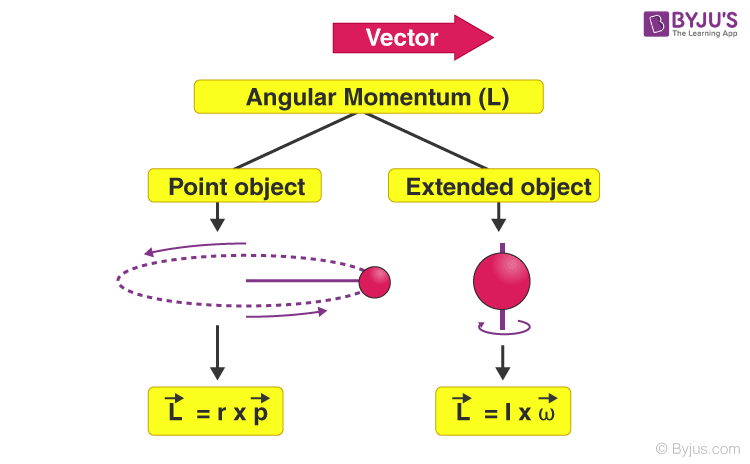# What is angular momentum?

Momentum is the product of mass and the velocity of the object. Any object moving with mass possesses momentum.

## Angular Momentum

The equivalent of linear momentum in rotational motion is angular momentum. It is the property of a rotating body given by the product of a moment of inertia and the angular velocity of a rotating object.
The Angular Momentum of a rotating body is proportional to its mass and to how rapidly it is turning.

• In addition, the angular momentum depends on how the mass is distributed relative to the axis of rotation: the further away the mass is located from the axis of rotation, the greater the angular momentum.
• A flat disk such as a record turntable has more angular momentum than a tall cylinder of the same mass and velocity of rotation.
• Like linear momentum, angular momentum is a vector quantity, and its conservation implies that the direction of the spin axis tends to remain unchanged.
• For this reason, the spinning top remains upright whereas a stationary one falls over immediately.

## Angular Momentum Formula

Angular momentum can be experienced by an object in two situations. They are:Where,

• $$\begin{array}{l}\vec{L}\end{array}$$
is the angular velocity
• r is the radius (distance between the object and the fixed point about which it revolves)
• $$\begin{array}{l} \vec{p}\end{array}$$
is the linear momentum.
• I is the rotational inertia.
• $$\begin{array}{l} \vec{\omega }\end{array}$$
is the angular velocity.

Examples

1. Ice-skater
2. Gyroscope

### Recommended Video### Articles to Explore:

2 (2)(1)(3)

#### Choose An Option That Best Describes Your Problem

Thank you. Your Feedback will Help us Serve you better.

#### 1 Comment

1. bharat kolhi

This web is a best option for learning for everyone .which are confused in some topics but do not wory .byju’s is here.